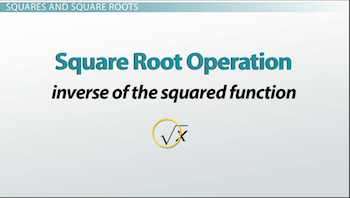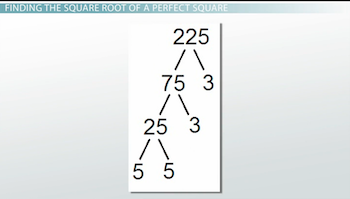# Evaluating Square Roots of Perfect Squares

Lesson Transcript
Instructor: Jennifer Beddoe

Jennifer has an MS in Chemistry and a BS in Biological Sciences.

Squares and square roots are inverse, or opposite, operations involving radicals. Learn how to determine the square root of perfect squares in this lesson.

## The Language of Math

Mathematics has its own special language. To understand and succeed in math, you need to be able to translate the language of math into English and back again. Just like you wouldn't move to France without knowing how to communicate at least the basics, you shouldn't venture into the study of math without knowing how to translate the terms you are using.

An error occurred trying to load this video.

Try refreshing the page, or contact customer support.

Coming up next: Estimating Square Roots

### You're on a roll. Keep up the good work!

Replay
Your next lesson will play in 10 seconds
• 0:05 The Language of Math
• 0:23 Squares and Square Roots
• 1:31 Perfect Square
• 1:56 Finding the Square…
• 4:37 Lesson Summary
Save Save

Want to watch this again later?

Timeline
Autoplay
Autoplay
Speed Speed

## Squares and Square Roots

The section of math dealing with radicals , or squares and square roots, is one place where knowing the translations of the terms is critical to understanding.

To square a number means to multiply that number by itself. It is notated by a superscript number 2 after the main number. It can also be written with a caret between the number being squared and the 2. When you see the carat symbol (^), you say that the number is squared.

For example:

3^2 is equal to 3*3 or 9

and

10^2 is equal to 10*10 or 100

The square root operation is the inverse of the squared function and is notated by this symbol:This means that finding the square root of a number is the same as finding the opposite of a number squared.

For example:

√100 is 10 because 10^2 is 100

and

√ 9 is 3 because 3^2 is 9

## Perfect Square

A number is a perfect square if it is an integer that is the square of another integer. An integer is a number that does not contain a fraction or a decimal.

For example:

5^2 is 25

So, 25 is a perfect square.

and

17^2 is 289, which means 289 is also a perfect square.

## Finding the Square Root of a Perfect Square

You can use a calculator to find the square root of a perfect square, but, if a calculator is not available, there is a way to calculate the square root.

Here are the steps to calculating the square root of a perfect square:

Step one: factor the number completely. An easy way to factor a number is by using a factor tree. A factor tree can be created by writing down the number you want to factor and drawing two lines coming down from that number. Then, write two factors of that number under the lines. Continue on until only prime numbers remain. A prime number is one that cannot be reduced any smaller. The purpose of the factor tree is so you can easily find the square root of large numbers if you don't have a calculator handy.

By looking at the factor tree below, you can see that the factors of 225 are 3 * 3 * 5 * 5.To unlock this lesson you must be a Study.com Member.

### Register to view this lesson

Are you a student or a teacher?